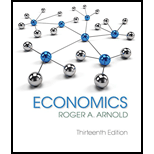# In long-run competitive equilibrium, P = MC = SRATC = LRATC. Because P = MR , we can write the preceding condition a s P = MR = MC = SRATC = LRATC. The condition thus consists of four parts: (a) P = MR , (b) MR = MC , (c) P = SRATC , and (d) SRATC = LRATC. Part (b) —MR = MC —is true because the perfectly competitive firm attempts to maximize profits, and that equation represents how it does so. What are the explanations for parts (a), (c), and (d)?### Economics (MindTap Course List)

13th Edition
Roger A. Arnold
Publisher: Cengage Learning
ISBN: 9781337617383### Economics (MindTap Course List)

13th Edition
Roger A. Arnold
Publisher: Cengage Learning
ISBN: 9781337617383

#### Solutions

Chapter
Section
Chapter 22, Problem 13QP
Textbook Problem

## Expert Solution

### Want to see the full answer?

Check out a sample textbook solution.See solution

### Want to see this answer and more?

Experts are waiting 24/7 to provide step-by-step solutions in as fast as 30 minutes!*

See Solution

*Response times vary by subject and question complexity. Median response time is 34 minutes and may be longer for new subjects.

Find more solutions based on key concepts
Show solutions
Use a production possibilities frontier to describe the idea of efficiency.

Principles of Microeconomics (MindTap Course List)

UNEVEN CASH FLOW STREAM a. Find the present values of the following cash flow streams at an 8% discount rate. b...

Fundamentals of Financial Management, Concise Edition (with Thomson ONE - Business School Edition, 1 term (6 months) Printed Access Card) (MindTap Course List)

What is the useful life of an asset?

College Accounting, Chapters 1-27 (New in Accounting from Heintz and Parry)